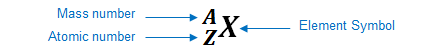# Problem: Four Fe2+ ions are key components of hemoglobin, the protein that transports oxygen in the blood. Assuming that these ions are 53Fe2+, how many protons and neutrons are present in each nucleus, and how many electrons are present in each ion?

###### FREE Expert Solution

We are being asked to determine the number of protons and neutrons present in each nucleus and the number of electrons present in each ion

An element can be represented as:You can determine the identity of the element using its atomic number:

Atomic number (Z):

represents the identity of the element
reveals the number of protons
in a neutral element:

# of protons = # of electrons

Mass number (A)

reveals the number of protons and neutrons

mass number = # of protons + # of neutrons

We will do the following steps

Step 1: Determine the number of protons

Step 2: Determine the number of neutrons

Step 3: Determine the number of electrons

Given: 53Fe2+

86% (471 ratings)###### Problem Details

Four Fe2+ ions are key components of hemoglobin, the protein that transports oxygen in the blood. Assuming that these ions are 53Fe2+, how many protons and neutrons are present in each nucleus, and how many electrons are present in each ion?

Frequently Asked Questions

What scientific concept do you need to know in order to solve this problem?

Our tutors have indicated that to solve this problem you will need to apply the Ions concept. You can view video lessons to learn Ions. Or if you need more Ions practice, you can also practice Ions practice problems.

What professor is this problem relevant for?

Based on our data, we think this problem is relevant for Professor Dunlavy's class at NMSU.

What textbook is this problem found in?

Our data indicates that this problem or a close variation was asked in Chemistry: An Atoms First Approach - Zumdahl Atoms 1st 2nd Edition. You can also practice Chemistry: An Atoms First Approach - Zumdahl Atoms 1st 2nd Edition practice problems.